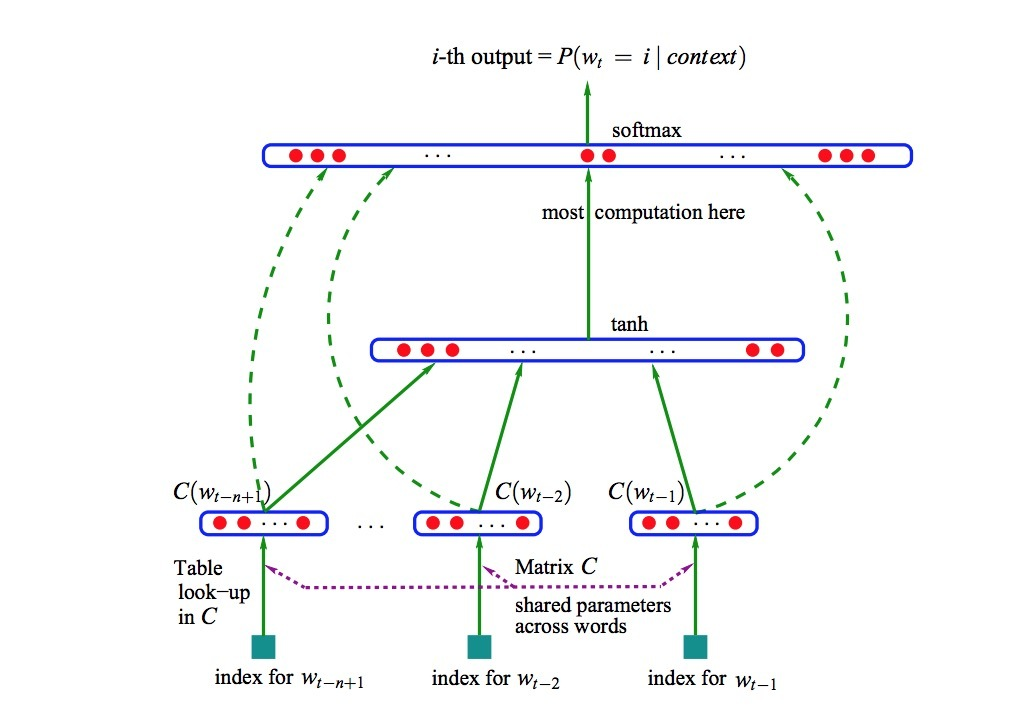NNLM介绍

January 03, 2016

Y Bengio在2003年发布了paper《A Neural Probabilistic Language Model》，即NNLM。我们来看一下这篇10多年前的paper中的主要内容：

1.介绍

$\hat{P}(W_1^T) = \prod\limits_{1}^{T} \hat{P}(w_t | w_t^{t-1})$

$\hat{P}(w_t | w_1^{t-1}) \approx \hat{P}(w_t | w_{t-n+1}^{t-1})$

1.1 使用分布式表示来解决维度灾难

• 1.将词表中的每个词与一个distributed word feature vector（在$R^m中$一个real-valued vector）进行关联。
• 2.采用在序列中的词的feature vectors来表示词序列的联合概率函数.
• 3.同时学习word vectors和概率函数的参数

feature vector可以表示词的不同方面：每个词与一个向量空间中的一个点（point）相关联。features的数目（比如：实验中采用m=30, 60或100）比词汇表的size要小很多。概率函数被表示成：给定之前的词，一个关于下一个词的条件概率乘积（例如：使用一个multi-layer NN来预测）。该函数具有这样的参数，它以最大化训练数据的log似然来进行迭代调参。feature vectors是通过学习得到的，但它们可以使用语义特征的先验知识进行初始化。

The cat is walking in the bedroom

A dog was running in a room

The cat is running in a room

A dog is walking in a bedroom

The dog was walking in the room

2.一个神经模型

$f(w_t, \cdots, w_{t-n+1}) = \hat{P}(w_t \mid w_1^{t-1})$

• 1.一个映射函数C，它将V的任意元素i映射到一个真实向量$C(i) \in R^m$。它表示与词典中每个词相关的分布式特征向量(distributed feature vectors)。实际上，C被表示成一个关于自由参数的$\mid V \mid \times m$的矩阵。
• 2.词上的概率函数，由C进行表示：对于在上下文中的词，$(C(w_{t-n+1}), \cdots, C(w_{t-1}))$，会使用一个函数g将一个关于feature vectors的输入序列映射到一个关于下一个词$w_t \in V$的条件概率分布上。g的输出是一个vector，它的第i个元素可以估计概率$\hat{P}(w_t = i \mid w_1^{t-1})$，如图1所示。
$f(i, w_{t-1}, \cdots, w_{t-n+1}) = g(i, C(w_{t-1}), \cdots, C(w_{t-n+1}))$$L = \frac{1}{T} \sum\limits_{t} log f(w_t, w_{t-1}, \cdots, w_{t-n+1}; \theta) + R(\theta)$

• 1.shared word features layer C：它是线性的
• 2.普通的双曲正切( hyperbolic tangent) hidden layer

$\hat{P}(w_t | w_{t-1}, \cdots, w_{t-n+1}) = \frac{e^{y_{w_t}}}{\sum\limits_{i} e^{y_i}}$

$y = b + W x + U tanh(d+Hx)$

…(1)

$x = (C(w_{t-1}), C(W_{t-2}), \cdots, C(W_{t-n+1}))$

$\theta = (b,d,W,U,H,C)$

$\theta \leftarrow \theta + \epsilon \frac{\partial log \hat{P}(w_t | w_{t-1}, \cdots, w_{t-n+1})}{\partial \theta}$

其它

http://www.jmlr.org/papers/volume3/bengio03a/bengio03a.pdf

Updated on

STAR算法介绍

PLE介绍

Published on March 04, 2021

DTS介绍

Published on January 02, 2021